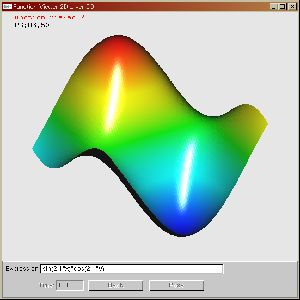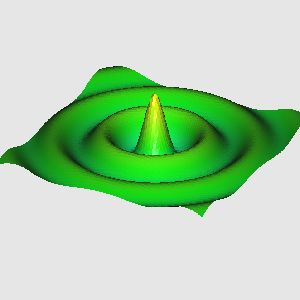| Top Page |

Function Viewer 2D     Last UpdateüF11/21/2006

 Introduction Enter a mathematical function, and this program will render the function in a 3D space. You can change viewpoint arbitrary with an intuitive operation. The function you input is a function of 'x', 'y' and 't'. 'x','y' represent the axis of coord the function is defined.And 't' represent time. For example the wave propagation in the direction of axis x is     sin(10*x-t) If the function you enter is a function of time, like previous example, you can stop or restart the time with "Pose" or "Play" button.The install is not requied.

Change Viewpoint

 Rotation Drug the middle button of mouse Parallel displacement ü¬ü½ü©ü® Zoom In / Out [Pg Up] Key for zoom in, [Pg Dn] Key for zoom out The distance of viewpoint [End] Key to switch the distance is finit / infinit If the distance id finit, press Shift Key with [Pg Up] Key for shorter distance, and Press Shift Key with [Pg Dn] Key for longer distance Reset [Home] Key initialize the Viewpoint

Interesting Functions

Please let me know if you find a interesting function.Ripple 0.05/(sqrt(x*x+y*y)+0.1)*sin(15*sqrt(x*x+y*y)-2*t)

Operands and Operators

Operands
 x Coordinate x y Coordinate y t Time PI circle ratio

Binary Operators
 + Addition - Substraction * Multiplication / Division ^ Exponation

Unary Operators
 +x sign + -x sign - abs(x) Absolute value floor(x) Maximum integral not exceed x sqrt(x) x^0.5 exp(x) e^x log(x) Natural Logalithm sin(x) Sine cos(x) Cosine

Update History
 11/16/2006 : Upload!!!! 11/19/2006 : Dealing with time dependent function

Please utilize this program for educational purpose.
Distributon of this program is free only for noncommercial use.
I don't compansate for any damage which may caused by this program.